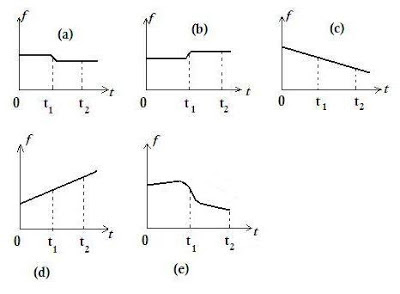## Pages

`“Life is like riding a bicycle.  To keep your balance you must keep moving.”–Albert Einstein`

## Friday, December 21, 2012

### AP Physics B - Multiple Choice Practice Questions on Doppler effect

In the post dated 9th April 2009 the essential points to be noted about Doppler effect were given. You may click here to access that post. All posts related to Doppler effect can be accessed by clicking on the label ‘Doppler effect’ below this post.

Today we shall discuss a few multiple choice practice questions involving Doppler effect:

(1) The figure above shows a car sounding its horn and moving with uniform velocity along a straight road. At time t = 0 the car is at position A and at time t = t1 the car moves past a stationary listener L. At time t = t2 the car is at position B. Which one among the following graphs best represents the variation of the frequency (pitch) of the horn with time as heard by the listener?When the car moves towards the listener the number of complete sound waves reaching the ear of the listener in one second is greater than the number reaching his ears when the car is at rest. The frequency of the horn as heard by the listener in this case is higher than the real requency of the horn. When the car moves away from the listener the number of complete sound waves reaching the ears of the listener in one second is less than the number of waves produced by the horn in one second.

[All the sound waves produced by the horn in one second.cannot reach the listener’s ears within one second since the car is moving away].

Therefore, the frequency of the horn as heard by the listener is less than the real frequency after the car moves past the listener.

The graph which best represents the variation of the frequency with time is (a).

(2) In the above question suppose the car is moving with uniform acceleration. Which one among the graphs will best represent the variation of the frequency (pitch) of the horn with time as heard by the listener?

In this case the pitch of the sound as heard by the listener will go on increasing when the car moves towards the listener since the relative velocity of the car with respect to the listener goes on increasing. After the car moves past the listener the pitch of the sound as heard by the listener will go on decreasing since the source of sound (horn) is moving away from the listener with continuously increasing velocity.

Therefore, the graph which best represents the variation of the frequency with time in this case is (e).

(3) A stationary police car at position A (Fig.) sounds its horn when the air is still and a listener L on the road hears the frequency of the note as n. A steady wind now blows continupusly from B to A with speed equal to 2 % of the speed of sound in air. What will be the frequency of the sound of  the siren as heard by the listener when the wind is blowing?

(a) 0.98 n

(b) 1.02 n

(c) n

(d) 0.99 n

(e) 1.01 n

The number of complete sound waves emitted per second by the siren will be n whether the wind is present or absent. Since the steady wind is blowing continuously a stationary listener will receive just n complete waves per second. The correct option is (c).

[If the sound source and listener are moving relative to each other, the listener will hear one shifted frequency (Doppler shift) in still air and a different shifted frequency if wind is blowing. However, if the source and the listener are at rest relative to each other, there is no Doppler shift when the wind is blowing. If you are confused, you may use the expression for the apparent frequency as heard by the listener:

Let the source S of sound (fig.) move with velocity vS, the listener move with velocity vL and the wind blow with velocity vw, all in the same direction as shown in the figure.

Wind→ w         S●→vS        L●→vL

The apparent frequency (n’) of sound is then given by

n’ = n(v + w vL)/ (v + w vS)

where n is the real frequency of the sound and v is the velocity of sound.

If vL and vS are zero we obtain n’ = n

When there is relative motion between the source and the listener, you will have to take the sign of the wind velocity w as positive, as given in the above expression, when the wind blows from source to the listener. If the wind blows from listener to source, the sign of w is to be taken as negative]

You will find more questions (with solution) in this section at